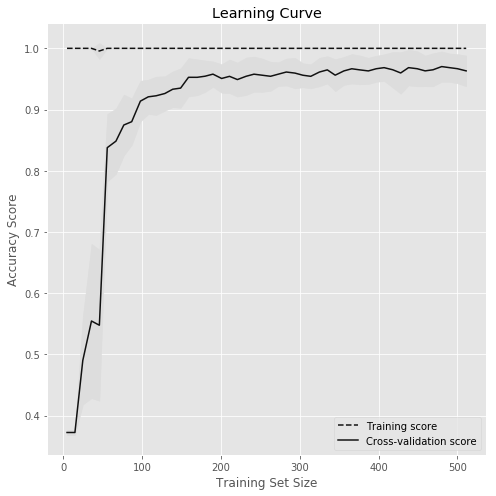How to evaluate XGBoost model with learning curves example 1?
MACHINE LEARNING RECIPES DATA CLEANING PYTHON DATA MUNGING PANDAS CHEATSHEET     ALL TAGS

# How to evaluate XGBoost model with learning curves example 1?

This recipe helps you evaluate XGBoost model with learning curves example 1

0
In :
```## How to evaluate XGBoost model with learning curves
def Snippet_188():
print()
print(format('Hoe to evaluate XGBoost model with learning curves','*^82'))

import warnings
warnings.filterwarnings("ignore")

import numpy as np
from xgboost import XGBClassifier
import matplotlib.pyplot as plt

plt.style.use('ggplot')

from sklearn import datasets
import matplotlib.pyplot as plt
from sklearn.model_selection import learning_curve

X = dataset.data; y = dataset.target

# Create CV training and test scores for various training set sizes
train_sizes, train_scores, test_scores = learning_curve(XGBClassifier(),
X, y, cv=10, scoring='accuracy', n_jobs=-1,
# 50 different sizes of the training set
train_sizes=np.linspace(0.01, 1.0, 50))

# Create means and standard deviations of training set scores
train_mean = np.mean(train_scores, axis=1)
train_std = np.std(train_scores, axis=1)

# Create means and standard deviations of test set scores
test_mean = np.mean(test_scores, axis=1)
test_std = np.std(test_scores, axis=1)

# Draw lines
plt.subplots(1, figsize=(7,7))
plt.plot(train_sizes, train_mean, '--', color="#111111",  label="Training score")
plt.plot(train_sizes, test_mean, color="#111111", label="Cross-validation score")

# Draw bands
plt.fill_between(train_sizes, train_mean - train_std, train_mean + train_std, color="#DDDDDD")
plt.fill_between(train_sizes, test_mean - test_std, test_mean + test_std, color="#DDDDDD")

# Create plot
plt.title("Learning Curve")
plt.xlabel("Training Set Size"), plt.ylabel("Accuracy Score"), plt.legend(loc="best")
plt.tight_layout(); plt.show()

Snippet_188()
```
```****************Hoe to evaluate XGBoost model with learning curves****************
```#### Relevant Projects

##### Machine Learning project for Retail Price Optimization
In this machine learning pricing project, we implement a retail price optimization algorithm using regression trees. This is one of the first steps to building a dynamic pricing model.

##### Choosing the right Time Series Forecasting Methods
There are different time series forecasting methods to forecast stock price, demand etc. In this machine learning project, you will learn to determine which forecasting method to be used when and how to apply with time series forecasting example.

##### Learn to prepare data for your next machine learning project
Text data requires special preparation before you can start using it for any machine learning project.In this ML project, you will learn about applying Machine Learning models to create classifiers and learn how to make sense of textual data.

##### Sequence Classification with LSTM RNN in Python with Keras
In this project, we are going to work on Sequence to Sequence Prediction using IMDB Movie Review Dataset​ using Keras in Python.

##### Music Recommendation System Project using Python and R
Machine Learning Project - Work with KKBOX's Music Recommendation System dataset to build the best music recommendation engine.

##### Human Activity Recognition Using Smartphones Data Set
In this deep learning project, you will build a classification system where to precisely identify human fitness activities.

##### Zillow’s Home Value Prediction (Zestimate)
Data Science Project in R -Build a machine learning algorithm to predict the future sale prices of homes.

##### German Credit Dataset Analysis to Classify Loan Applications
In this data science project, you will work with German credit dataset using classification techniques like Decision Tree, Neural Networks etc to classify loan applications using R.

##### Natural language processing Chatbot application using NLTK for text classification
In this NLP AI application, we build the core conversational engine for a chatbot. We use the popular NLTK text classification library to achieve this.

##### Customer Churn Prediction Analysis using Ensemble Techniques
In this machine learning churn project, we implement a churn prediction model in python using ensemble techniques.